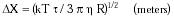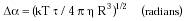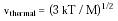Nanomedicine, Volume I: Basic Capabilities

Robert A. Freitas Jr., Nanomedicine, Volume I: Basic Capabilities, Landes Bioscience, Georgetown, TX, 1999

3.2.1 Brownian Motion

A particle suspended in a fluid is subjected to continuous collisions, from all directions, with the surrounding molecules. If the velocities of all molecules were the same all the time, the particle would experience no net movement. However, molecules do not have a single velocity at a given temperature, but rather have a distribution of velocities of varying degrees of probability. Thus from time to time, a suspended particle receives a finite momentum of unpredictable direction and magnitude. The velocity vector of the particle changes continuously, resulting in an observable random zigzag movement called Brownian motion.

Einstein385 approximated the RMS (root mean square) displacement of a particle of radius R suspended in a fluid of absolute viscosity h and temperature T, after an observation period t, as:{Eqn. 3.1}

where k = 1.381 x 10-23 joule/kelvin (K) or 0.01381 zJ/K (Boltzmann's constant). Particles under bombardment also experience a rotational Brownian motion around randomly oriented axes, with the RMS angle of rotation:{Eqn. 3.2}

although for t < tmin = M / 15 p h R, where M is particle mass (see below), rotation is ballistic.

In human blood plasma, with h = 1.1 centipoise (1.1 x 10-3 kg/m-sec) and T = 310 K, a spherical 1-micron diameter nanodevice (R = 0.5 micron) translates ~1 micron in 1 sec (vbrownian ~ 10-6 m/sec) or ~8 microns (~the width of a capillary) after 77 sec (vbrownian ~ 107 m/sec), and rotates once in ~16 sec (tmin = 2 x 10-8 sec). In the same environment, a rigid 10-nm particle (roughly the diameter of a globular protein) would translate ~8 microns in one second (vbrownian ~ 10-5 m/sec) while rotating ~250 times, due to Brownian motion (tmin = 2 x 10-12 sec).

The instantaneous thermal velocity over one mean free path, the average distance between collisions, is much higher than the net Brownian translational velocity would suggest. For a particle of mass M = 4/3 p r R3 with mean (working) density r, the mean thermal velocity is:{Eqn. 3.3}

At T = 310 K, a spherical 1-micron diameter nanodevice of normal density (e.g., taking r ~ rH2O = 993.4 kg/m3 to minimize ballasting requirements; Section 10.3.6) has vthermal ~ 5 x 10-3 m/sec; for a spherical 10-nm diameter protein with r ~ 1500 kg/m3, vthermal ~ 4 m/sec.

Last updated on 7 February 2003